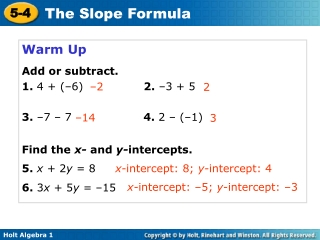DownloadDownload PresentationWarm Up Add or subtract. 1. 4 + (–6) 2. –3 + 5

# Warm Up Add or subtract. 1. 4 + (–6) 2. –3 + 5

Télécharger la présentation## Warm Up Add or subtract. 1. 4 + (–6) 2. –3 + 5

- - - - - - - - - - - - - - - - - - - - - - - - - - - E N D - - - - - - - - - - - - - - - - - - - - - - - - - - -
##### Presentation Transcript

1. Warm Up Add or subtract. 1. 4 + (–6) 2. –3 + 5 3. –7 – 7 4. 2 – (–1) –2 2 –14 3 Find the x- and y-intercepts. 5. x + 2y = 8 6. 3x + 5y = –15 x-intercept: 8; y-intercept: 4 x-intercept: –5; y-intercept: –3

2. Objective Find slope by using the slope formula.

3. In Lesson 5-3, slope was described as the constant rate of change of a line. You saw how to find the slope of a line by using its graph. There is also a formula you can use to find the slope of a line, which is usually represented by the letter m. To use this formula, you need the coordinates of two different points on the line.

4. The slope of the line that contains (2, 5) and (8, 1) is . Example 1 Find the slope of the line that contains (2, 5) and (8, 1). Use the slope formula. Substitute (2, 5) for (x1, y1) and (8, 1) for (x2, y2). Simplify.

5. Example 2 Find the slope of the line that contains (–2, –2) and (7, –2). Use the slope formula. Substitute (–2, –2) for (x1, y1) and (7, –2) for (x2, y2). Simplify. = 0 The slope of the line that contains (–2, –2) and (7, –2) is 0.

6. Example 3 Find the slope of the line that contains (5, –7) and (6, –4). Use the slope formula. Substitute (5, –7) for (x1, y1) and (6, –4) for (x2, y2). Simplify. = 3 The slope of the line that contains (5, –7) and (6, –4) is 3.

7. Substitute for (x1, y1) and for (x2, y2) and simplify. The slope of the line that contains and is 2. Example 4 Find the slope of the line that contains and Use the slope formula.

8. Sometimes you are not given two points to use in the formula. You might have to choose two points from a graph or a table. Directions: Find two points on the graph or table and determine the slope using the formula.

9. Example 5 Let (0, 2) be (x1, y1) and (–2, –2) be (x2, y2). Use the slope formula. Substitute (0, 2) for (x1, y1) and (–2, –2) for (x2, y2). Simplify.

10. Substitute (0, 1) for and (–2, 5) for . Example 6 Step 1 Choose any two points from the table. Let (0, 1) be (x1, y1) and (–2, 5) be (x2, y2). Step 2 Use the slope formula. Use the slope formula. Simplify. The slope equals −2

11. Example 7 Let (–2, 4) be (x1, y1) and (0, –2) be (x2, y2). Use the slope formula. Substitute (–2, 4) for (x1, y1) and (0, –2) for (x2, y2). Simplify.

12. Example 8 Step 1 Choose any two points from the table. Let (0, 0) be (x1, y1) and (–2, 3) be (x2, y2). Step 2 Use the slope formula. Use the slope formula. Substitute (0, 0) for (x1, y1) and (–2, 3) for (x2, y2). Simplify

13. Remember that slope is a rate of change. In real-world problems, finding the slope can give you information about how a quantity is changing.

14. Example 9 The graph shows the average electricity costs (in dollars) for operating a refrigerator for several months. Find the slope of the line. Then tell what the slope represents. Step 1 Use the slope formula.

15. So slope represents in units of . Example 9 Continued Step 2 Tell what the slope represents. In this situation yrepresents the cost of electricity and xrepresents time. A slope of 6 mean the cost of running the refrigerator is a rate of 6 dollars per month.

16. Lesson Summary 1. Find the slope of the line that contains (5, 3) and (–1, 4). 2. Find the slope of the line. Then tell what the slope represents. 50; speed of bus is 50 mi/h